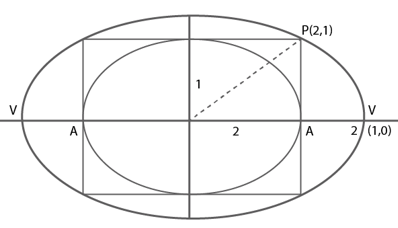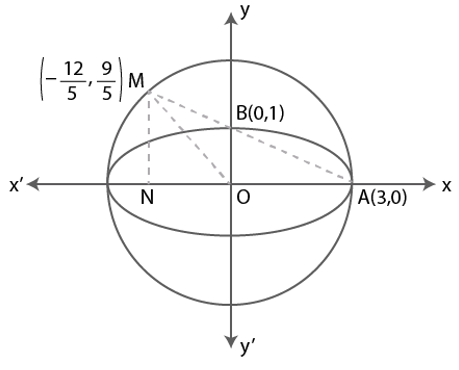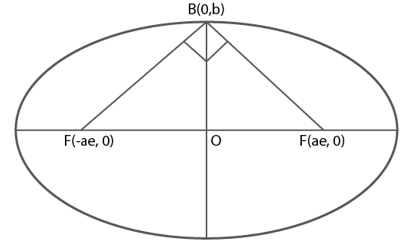# JEE Ellipse Previous Year Questions With Solutions

In conic section, ellipse is a geometrical shape of which every point is the same total distance from the two fixed points. Previous Year Questions With Solutions on Ellipse is a very important resource for JEE aspirants. While studying this section students will come across questions asked in previous year JEE examinations. In order to help students to develop better problems skills we are offering free solutions on this page along with the pdf to study offline. These solutions will help students understand the topics of ellipse more clearly. BYJU’S provide all chapter-wise previous year questions with solutions to help students prepare well for exam and clear doubts. However, some of the problems given in the chapter include: derivation of equation of the ellipse under various conditions, latus rectums of ellipse, area of inscribed figures, foci of the ellipse, tangent to ellipse and many more.

### Important Points:

 1. Define Ellipse: An ellipse is the set of all points in a plane such that the sum of the distances of each point from two fixed points A and A’ in the plane is constant. 2. Focus: One of the two fixed points that define an ellipse. 3. Eccentricity: The result obtained by dividing the distance between the foci by the longest distance across an ellipse (the major axis). Note: An ellipse with zero eccentricity, that is circle. 4. An ellipse may be drawn by the concentric or auxiliary circles methods.

## JEE Past Year Questions With Solutions on Ellipse

Question 1: The normal at a point P on the ellipse x2 + 4y2 = 16 meets x-axis at Q. If M is the mid point of the line segment PQ, then the locus of M intersects the latus rectums of the given ellipse at the point

(a) ($\pm \frac{3 \sqrt 5}{2}, \pm \frac{2}{7}$)

(b) ($\pm \frac{3 \sqrt 5}{2}, \pm \frac{\sqrt{19}}{4}$)

(c) ($\pm 2 \sqrt {3}, \pm \frac{1}{7}$)

(d) ($\pm 2 \sqrt {3}, \pm \frac{4 \sqrt{3}}{7}$)

Solution:

Equation of ellipse x2 + 4y2 = 16

or $\frac{x^2}{16} + \frac{y^2}{4} = 1$

Now,

4x sec θ – 2y cosec θ = 12 and x = 3 cos θ

Coordinates of point Q: (3 cos θ, 0)

Also, 2h = 7 cos θ and 2k = 2 sin θ

=> $\frac{4x^2}{49} + \frac{y^2}{1} = 1$

Latus rectum = x = 2√3

and y = ± 1/7

Therefore, required point is (± 2√3, ±1/7)

Question 2: The ellipse x2 + 4y2 = 4 is inscribed in a rectangle aligned with the coordinate axes, which in turn in inscribed in another ellipse that passes through the point (4, 0). Find the equation of the ellipse.

Solution:

Given equation of ellipse : x2 + 4y2 = 4

=> x2/4 + y2/1 = 1

Here a = 2 and b = 1

We know the general equation of ellipse is x2/ a2+ y2/b2 = 14/16 + 1/b2

=> b2 = 4/3

Therefore, x2/16 + y2/(4/3) = 1

=> x2/16 + 3y2/4 = 1

=> x2 + 12 y2 = 16

Question 3: The line passing through the extremity A of the major axis and extremity B of the minor axis of the ellipse x2 + 9y2 = 9 meets its auxiliary circle at the point M. Find the area of the triangle with vertices at A, M and the origin O.Solution:

Equation of AM: y = -1/3(x + 3)

or x + 3y + 3 = 0, Slope of line = -1/3

So, y – 1 = -1/3(x – 0) => 3y + x – 3 = 0

=> Equation of AM is 3y + x – 3 = 0, which is chord of auxiliary circle, x2 + 9y2 = 9.

Coordinates of point A(3,0) and B(0, 1).

Distance of AM from the origin = |(0+0-3)/(√(9+1)| = 3/√10

The equation of an auxiliary circle of an ellipse is x2 + y2 = 9

From figure, line AM cuts auxiliary circle at M.

By solving these equations, we have M(-12/5, 9/5) co-ordinates of point M.

Area of triangle AOM = 1/2 |0(0 – 9/5) + 3(9/5 – 0) – 12/5(0-0)|

= 27/10

Question 4: If 3x + 4y = 12√2 is a tangent to the ellipse x2/a2 + y2/9 = 1, for some a Є R then the distance between the foci of the ellipse is:

(a) 2√5                 (b) 2√7                           (c) 2√2                  (d) 4

Solution:

Here 3x + 4y = 12√2 is the tangent to ellipse.

=> y = -3x/4 + 3√2 ..(1)

We know, the equation of tangent to ellipse x2/a2 + y2/b2 = 1 is y = mx + √(a2m2 + b2)

Here b2 = 9

So, equation of tangent to given ellipse is y = mx + √(a2m2 + 9)

Now, using (1), we have

m = -3/4 and √(a2m2 + 9) = 3√2

=> (a2m2 + 9) = 18

=> (a2(-3/4)2 + 9) = 18

=> a2 x 9/16 = 9

=> a = 4

Now, e2 = 1 – b2/a2 = 1 – 9/16 = 7/16

Or e = √7/4

The distance between foci is 2ae = 2 x 4 x √7/4 = 2√7

Question 5: An ellipse has OB as semi minor axis, F and F’ its focii and the angle FBF’ is a right angle. Then the eccentricity of the ellipse is

(a) 1/√2                  (b) 1/2                       (c) 1/4                        (d) 1/√3

Solution:The angle FBF’ is a right angle.

Therefore,

$(\sqrt{a^2e^2 + b^2)^2} + (\sqrt{a^2e^2 + b^2)^2} = (2ae)^2$

=> 2(a2e2 + b2) = 4a2e2

=> e2 = b2/a2

We know, e2 = 1 – b2/a2 = 1 – e2

=> 2e2 = 1

or e = 1/√2

Question 6: Find the equation of the ellipse whose axes are the axes of coordinates and which passes through the point (-3, 1) and has eccentricity √(2/5)

Solution:

Given, eccentricity = √(2/5)

e2 = 2/5

we know, e2 = 1 – b2/a2

=> b2/a2 = 1 – 2/5 = 3/5

=> x2/5k + y2/3k = 1, this equation passes through the point (-3, 1)

=> (9/5) + (1/3) = k

Or k = 32/15

Therefore, required equation of ellipse is 3x2/32 + 5y2/32 = 1

Question 7: The eccentricity of an ellipse, with its centre at the origin is 1/2. If one of the directrices is x = 4, then what will be the equation of the ellipse.

Solution:

We know, equation of directrix is x = a/e = 4

=> a = 2

Now, b2 = a2 (1 – e2)

=> b2 = 3

Therefore, the equation of ellipse is 3x2 + 4y2 = 12

Question 8: Tangents are drawn from the point P(3, 4) to the ellipse x2/9 + y2/4 = 1 touching the ellipse at points A and B

The coordinates of A and B are

(a) (3, 0) and (0, 2)

(b) (-8/5, 2√26/15) and (-9/8, 8/5)

(c) (-8/5, 2√16/15) and (0, 2)

(d) (3,0) and (-9/5, 8/5)

Solution:

Given equation of ellipse is x2/9 + y2/4 = 1 …(1)

The equation of chord is

xx1/a2 + yy1/b2 = 1

3x/9 + 4y/4 = 1

=> x + 3y – 3 = 0

=> x = 3(-y + 1)

Now,

(1) =>

$\frac{9(-y+1)^2}{9} + \frac{y^2}{4} = 1$

=> y2 – 2y + 1 + y2/4 = 1

=> 5y2 – 2y = 0

=> y(5y/4 – 2) = 0

=> y = 0 or y = 8/5

So, x = 3 – 3y

At y = 0 => x = 3

At y = 8/5 => x = -9/5

Therefore, the required coordinates are (3, 0) and (-9/5, 8/5)

Question 9: In an ellipse, the distance between its foci is 6 and minor axis is 8. Then its eccentricity is

(a) 3/5                     (b) ½                    (c) 4/5                  (d) 1/√5

Solution:

In an ellipse, the distance between its foci is 6 and minor axis is 8

The equation of ellipse,

$\frac{x^2}{a^2} + \frac{y^2}{b^2} = 1, \; \; a^2 > b^2$

and b2/a2 = 1 – e2 …(1)

Therefore, ae/b = 3/4

=> e2 = 9/16 x b2/a2

Using (1), we have

e = 3/5

Question 10: If the distance between the foci of an ellipse is 6 and the distance between its directrices is 12, then the length of its latus rectum is

(a) 2√3                   (b) √3                      (c) 3/√2                       (d) 3√2

Solution:

Let the equation of ellipse be x2/a2 + y2/b2 = 1 (a > b)

Now, 2ae = 6 and 2a/e = 12

=> ae = 3 and a/e = 6

=> a2 = 18

=> a2e2 = c2 = a2 – b2 = 9

=> b2 = 9

Length of latus rectum = 2b2/a

= 18/√18

= 3√2

Solve More Related Questions:

Conic Sections Previous Year Questions With Solutions

Hyperbola Previous Year Questions With Solutions

Parabola Previous Year Questions With Solutions

Circle Previous Year Questions With Solutions